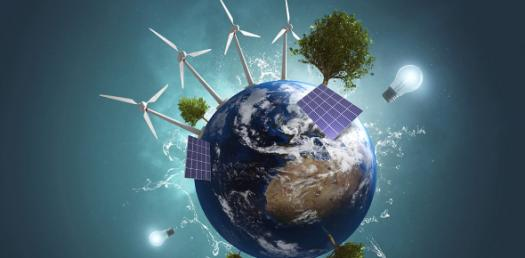# A Trivia Quiz On Temperature And Thermal Energy?

20 Questions | Total Attempts: 136SettingsThings are heating up in this quiz, as we take a look at the topics of temperature, which you know all about when you check the thermostat or have to use a thermometer, as well as thermal energy – the energy produced by heat. What do you know about the two? Take the quiz and we’ll find out!

• 1.
The lowest temperature possible in nature is
• A.

0 degrees C

• B.

-273 degrees C

• C.

4 K

• 2.
A volume of air has a temperature of 0 degrees Celsius. An equal volume of air that is twice as hot has a temperature of
• A.

0 degrees C

• B.

64 degrees C

• C.

100 degrees C

• D.

None of the above choices are correct

• 3.
To wholly convert a given amount of heat energy into mechanical energy is
• A.

Possible using a steam engine

• B.

Possible using an atomic reactor

• C.

Possible using a simple machine

• D.

Impossible regardless of the technique used

• 4.
To totally convert a given amount of mechanical energy into heat is
• A.

Possible

• B.

Impossible

• C.

• 5.
The greater the difference in temperature between the input reservoir and the output reservoir for a heat engine, the
• A.

Greater the efficiency

• B.

Less the efficiency

• C.

Neither efficiency of a heat engine doesn't depend on temperature difference

• 6.
The first law of thermodynamics is a restatement of the
• A.

Principle of entropy

• B.

• C.

Carnot cycle

• D.

Conservation of energy

• E.

None of these

• 7.
Chinook winds tend to
• A.

Warm an environment

• B.

Cool an environment

• C.

Cool deserts at night

• D.

Warm deserts at sunrise

• 8.
• A.

Less entropy

• B.

More entropy

• C.

No entropy

• 9.
A temperature inversion occurs when the upper layers of air are
• A.

Warmer than the lower regions of air

• B.

Cooler than the lower regions of air

• C.

At the same temperature as lower regions of air

• 10.
Entropy measures
• A.

Temperature at constant pressure

• B.

Temperature at constant volume

• C.

Temperature as pressure increases

• D.

Temperature as volume increases

• E.

Messiness

• 11.
Entropy is closely related to the
• A.

1st law of thermodynamics

• B.

2nd law of thermodynamics

• C.

Both of these

• D.

Neither of these

• 12.
• A.

Warmer still

• B.

Cooler

• C.

Neither warmer nor cooler

• 13.
The earth's atmosphere gets most of its heat
• A.

Near the surface of the Earth

• B.

At high altitudes closer to the sun

• C.

From the Earth's core

• 14.
Industrialization on Earth eventually heats our surroundings to temperatures that are higher than usual. Industrialization in space habitats using exclusively solar power eventually will thermally pollute the solar
• A.

True

• B.

False

• C.

Cannot be determined without additional information

• 15.
• A.

Only in the atmosphere

• B.

Only in the oceans

• C.

Only in the earth's mantle

• D.

In both the atmosphere and the oceans

• E.

In the atmosphere, oceans and the earth's mantle

• 16.
When an ideal gas is subjected to an adiabatic process
• A.

No work is done on the gas

• B.

The temperature of the gas does not change

• C.

The internal energy of the gas does not change

• D.

Choices A, B, and C are all true

• E.

None of the above choices are true

• 17.
During an adiabatic compression of an ideal gas
• A.

The internal energy of the gas remains constant

• B.

The temperature of the gas does not change

• C.

No heat is supplied to or removed from the gas

• D.

No work is done on the gas

• E.

None of the above choices are true

• 18.
A process which takes place at constant temperature is
• A.

• B.

An isothermal process

• C.

Impossible

• 19.
A quantity of water has more entropy when it is
• A.

Frozen ice

• B.

Boiling

• C.

At room temperature

• 20.
Entropy can be
• A.

Created but not destroyed

• B.

Neither created nor destroyed

• C.

Sometimes destroyed but never created

Related TopicsBack to top